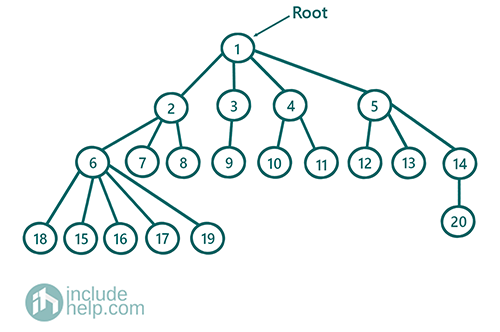# Find All Root to Leaf Paths in an N-array Tree

In this article, we are going to discuss how to find all root to leaf paths in an n-array tree?
Submitted by Radib Kar, on July 31, 2020

Prerequisite: N-array TreeIn the above example, all the root to leaf paths are:

[1->2->6->15]
[1->2->6->16]
[1->2->6->17]
[1->2->6->18]
[1->2->6->19]
Etcs.

We can find all root to leaf paths by depth-first search traversals.

The algorithm is:

We will store the path as a string, so each path will be each string and to store the paths as a string of vector.

```void  all_root_to_leaf(TreeNode root, string current_path, vector<string> paths)
if(root is a leaf)
Append the root value to string current_path
End if

Append the root value to string current_path
for each child of this root:
Recursively call the function all_root_to_leaf(child, current_path, paths)
End for
End Function
```

Below is the implementation for the above algorithm:

```#include <bits/stdc++.h>
using namespace std;

class TreeNode {
public:
int val;
vector<TreeNode*> children;
TreeNode(int v)
{
val = v;
}
TreeNode(int v, int n)
{
val = v;
children = vector<TreeNode*>(n, NULL);
}
};

//recursive function to find all store to leaf paths
void all_root_to_leaf_paths(TreeNode* root, string s, vector<string>& paths)
{
if (!root)
return;
//leaf node
if (root->children.size() == 0) {
//append root->val to the current path
s += to_string(root->val);
paths.push_back(s);
return;
}

s += to_string(root->val) + "->";

for (int i = 0; i < root->children.size(); i++) {
all_root_to_leaf_paths(root->children[i], s, paths);
}
}

int main()
{
TreeNode* root = new TreeNode(1, 4);

root->children = new TreeNode(2, 3);
root->children = new TreeNode(3, 1);
root->children = new TreeNode(4, 2);
root->children = new TreeNode(5, 3);

root->children->children = new TreeNode(6, 5);
root->children->children = new TreeNode(7);
root->children->children = new TreeNode(8);

root->children->children = new TreeNode(9);

root->children->children = new TreeNode(10);
root->children->children = new TreeNode(11);

root->children->children = new TreeNode(12);
root->children->children = new TreeNode(13);
root->children->children = new TreeNode(14, 1);

root->children->children->children = new TreeNode(15);
root->children->children->children = new TreeNode(16);
root->children->children->children = new TreeNode(17);
root->children->children->children = new TreeNode(18);
root->children->children->children = new TreeNode(19);

root->children->children->children = new TreeNode(20);
vector<string> paths;
all_root_to_leaf_paths(root, "", paths);

cout << "All root to leaf paths are:\n";
for (auto it : paths)
cout << it << "\n";

return 0;
}
```

Output:

```All root to leaf paths are:
1->2->6->15
1->2->6->16
1->2->6->17
1->2->6->18
1->2->6->19
1->2->7
1->2->8
1->3->9
1->4->10
1->4->11
1->5->12
1->5->13
1->5->14->20
```

To show how this is working, we can dry-run up to a few steps.

```We define vector<string> arr
So at the main function, we call all_root_to_leaf_paths (root, "", arr)
So call to   all_root_to_leaf_paths (1, "", arr)
------------------------------------------------

all_root_to_leaf_paths(1, "", arr)
it's not NULL
It's not a leaf node
Append root->val to current path("")
So current path now "1"+"->"
Now for each child,
we will call all_root_to_leaf_paths(child of 1, "1", arr)
Say for example the child is 2,
so it will call all_root_to_leaf_paths(2, "1->", arr)
------------------------------------------------

all_root_to_leaf_paths(2, "1->", arr)
it's not NULL
It's not a leaf node
Append root->val to current path("1->")
So current path now "1"+"->"+"2"+"->"
Now for each child,
we will call all_root_to_leaf_paths(child of 2, "1", arr)
Say for example the child is 6,
so it will call all_root_to_leaf_paths(6, "1->2->", arr)
------------------------------------------------

all_root_to_leaf_paths(6, "1->2->", arr)
it's not NULL
It's not a leaf node
Append root->val to current path("1->2->")
So current path now "1->2->6->"
Now for each child
we will call all_root_to_leaf_paths(child of 6, "1->2->6->", arr)
Say for example the child is 6,
so it will call all_root_to_leaf_paths(15, "1->2->6->", arr)
------------------------------------------------

all_root_to_leaf_paths(15, "1->2->6->", arr)
it's not NULL
It's a leaf node
Append root->val to current path("1->2->6->")
So current path now "1->2->6->15"
```

Since it's the leaf node, we add the current path to vector<string> arr and return. Remember we passed a reference to the vector so it will store all the paths.

In this way, after performing all the recursion we will have all the root to leaf paths stored.

What's New

Top Interview Coding Problems/Challenges!

Languages: » C » C++ » C++ STL » Java » Data Structure » C#.Net » Android » Kotlin » SQL
Web Technologies: » PHP » Python » JavaScript » CSS » Ajax » Node.js » Web programming/HTML
Solved programs: » C » C++ » DS » Java » C#
Aptitude que. & ans.: » C » C++ » Java » DBMS
Interview que. & ans.: » C » Embedded C » Java » SEO » HR
CS Subjects: » CS Basics » O.S. » Networks » DBMS » Embedded Systems » Cloud Computing
» Machine learning » CS Organizations » Linux » DOS
More: » Articles » Puzzles » News/Updates